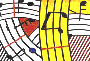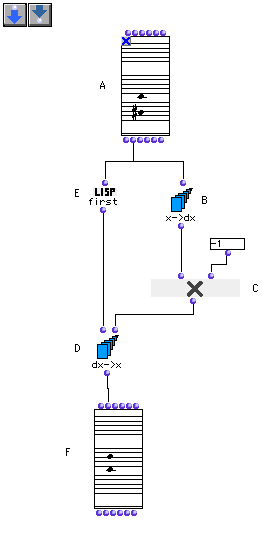Tutorial 4 - Inversion of an interval (II)

Topics

Note inversion using arithmetical series functions.

Functions used

Using CHORD,x->dx, dx->x, om*, and list-max.Description

This patch returns the inversion of an interval in the CHORD box (A) starting from C3. This example is a variant of tutorial2 .

Patch structure

A: Open the CHORD box (A) by clicking in the center of the box. Edit your own interval starting from the desired note (here C3) in the "order" mode. Then close the CHORD box and lock it by typing 'b'.

B: The x->dx box returnsthe intervals between a series of elements in a list. Here it will return the interval between the two notes in the CHORD box (A).

Note: It is important to rememeber that CHORD will return a list of notes in the same order you have edited these notes. If you have edited first the upper note of the interval x->dx will return a negative integer, meaning that the interval is descending as it is the case of our example. If on the contrary you edit the lower note first x->dx will return a positive integer representing an ascending one.

C: To invert the interval, we will multiply the output of x->dx by -1 using om*.

D: The dx->x box returns a list starting from a given number (first argument) incrementing step by step following a list of intervals (second argument).

E: We will use the first function (E) which will return the first note of our interval inside the CHORD box (A) (which is the firstedited note). The second argument of dx->x will be the inverted interval coming from om* (C)

F: Evaluate the CHORD box (F) and see the result. It will return the inverted interval.